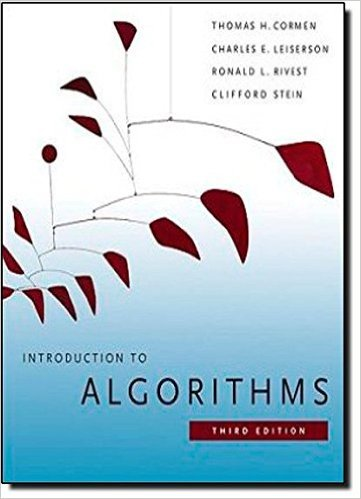×
Get Full Access to Introduction To Algorithms - 3 Edition - Chapter 14 - Problem 14-2
Get Full Access to Introduction To Algorithms - 3 Edition - Chapter 14 - Problem 14-2

×

# Josephus permutation We define the Josephus problem asISBN: 9780262033848 130

## Solution for problem 14-2 Chapter 14

Introduction to Algorithms | 3rd Edition

• Textbook Solutions
• 2901 Step-by-step solutions solved by professors and subject experts
• Get 24/7 help from StudySoup virtual teaching assistantsIntroduction to Algorithms | 3rd Edition

4 5 1 324 Reviews
30
4
Problem 14-2

Josephus permutation We define the Josephus problem as follows. Suppose that n people form a circle and that we are given a positive integer m n. Beginning with a designated first person, we proceed around the circle, removing every mth person. After each person is removed, counting continues around the circle that remains. This process continues until we have removed all n people. The order in which the people are removed from the circle defines the .n; m/-Josephus permutation of the integers 1; 2; : : : ; n. For example, the .7; 3/-Josephus permutation is h3; 6; 2; 7; 5; 1; 4i. a. Suppose that m is a constant. Describe an O.n/-time algorithm that, given an integer n, outputs the .n; m/-Josephus permutation. b. Suppose that m is not a constant. Describe an O.n lg n/-time algorithm that, given integers n and m, outputs the .n; m/-Josephus permutation.

Step-by-Step Solution:
Step 1 of 3

"-lc-tdlcl.}o trJ.e\trAt : 4 c> JE o flA,r r ('i- i tkL C L\*.:i,,\ l/1i\tltx -)K{'_rfc\\V\\ tj ' i\'t'[*) \.3 ittlt'c.>,[vl, { "[*) (a '\X):i,r-\i"",nq,- * i'f' E'f i 13 dq-crurr.r^3, { " [*] \o*ileg. !r,,n ..i i). Ccnftnx-uQ ofr {"* ro \c;{vct \ , i{ '{'r ib (ntrac,,bir} o'J.e.{ rft^-" \fihPrve..q + ,'{ ,: Ccnfctv{- cfe'r,^:fOn {* ift\enrJ r f, -(' iS det{ocr.:rir.;\ }J a/ {.,t- tn\arv&-t d fr \t t',[r) 3E-t*: Looc

Step 2 of 3

Step 3 of 3

##### ISBN: 9780262033848

Since the solution to 14-2 from 14 chapter was answered, more than 324 students have viewed the full step-by-step answer. The answer to “Josephus permutation We define the Josephus problem as follows. Suppose that n people form a circle and that we are given a positive integer m n. Beginning with a designated first person, we proceed around the circle, removing every mth person. After each person is removed, counting continues around the circle that remains. This process continues until we have removed all n people. The order in which the people are removed from the circle defines the .n; m/-Josephus permutation of the integers 1; 2; : : : ; n. For example, the .7; 3/-Josephus permutation is h3; 6; 2; 7; 5; 1; 4i. a. Suppose that m is a constant. Describe an O.n/-time algorithm that, given an integer n, outputs the .n; m/-Josephus permutation. b. Suppose that m is not a constant. Describe an O.n lg n/-time algorithm that, given integers n and m, outputs the .n; m/-Josephus permutation.” is broken down into a number of easy to follow steps, and 149 words. This textbook survival guide was created for the textbook: Introduction to Algorithms, edition: 3. Introduction to Algorithms was written by and is associated to the ISBN: 9780262033848. The full step-by-step solution to problem: 14-2 from chapter: 14 was answered by , our top Engineering and Tech solution expert on 11/10/17, 05:55PM. This full solution covers the following key subjects: Josephus, Permutation, circle, suppose, Person. This expansive textbook survival guide covers 35 chapters, and 151 solutions.

Unlock Textbook Solution